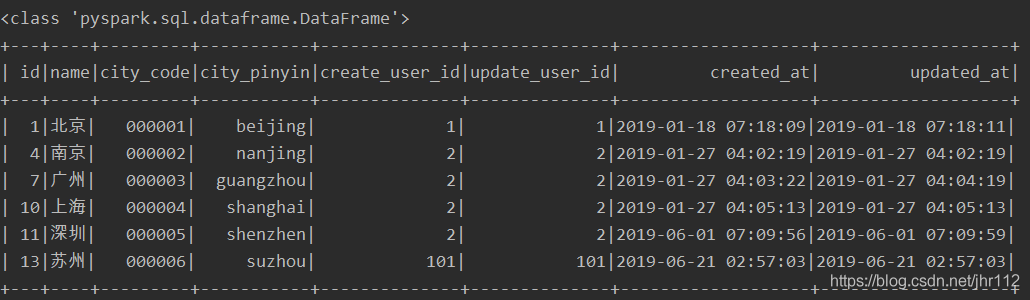# pyspark對Mysql數據庫進行讀寫的實現

pyspark是Spark對Python的api接口，可以在Python環境中通過調用pyspark模塊來操作spark，完成大數據框架下的數據分析與挖掘。其中，數據的讀寫是基礎操作，pyspark的子模塊pyspark.sql 可以完成大部分類型的數據讀寫。文本介紹在pyspark中讀寫Mysql數據庫。

### 1 軟件版本

• win10 64bit
• java 13.0.1
• spark 3.0
• python 3.8
• pyspark 3.0
• pycharm 2019.3.4

### 2 環境配置

pyspark連接Mysql是通過java實現的，所以需要下載連接Mysql的jar包。### 3 讀取Mysql

```from pyspark.sql import SQLContext, SparkSession

if __name__ == '__main__':
# spark 初始化
spark = SparkSession. \
Builder(). \
appName('sql'). \
master('local'). \
getOrCreate()
# mysql 配置(需要修改)
prop = {'user': 'xxx',
'driver': 'com.mysql.cj.jdbc.Driver'}
# database 地址(需要修改)
url = 'jdbc:mysql://host:port/database'
# 讀取表
# 打印data數據類型
print(type(data))
# 展示數據
data.show()
# 關閉spark會話
spark.stop()```
• 註意點：
• `prop`參數需要根據實際情況修改，文中用戶名和密碼用xxx代替瞭，`driver`參數也可以不需要；
• `url`參數需要根據實際情況修改，格式為`jdbc:mysql://主機:端口/數據庫`
• 通過調用方法`read.jdbc`進行讀取，返回的數據類型為spark DataFrame；### 4 寫入Mysql

```import pandas as pd
from pyspark import SparkContext
from pyspark.sql import SQLContext, Row

if __name__ == '__main__':
# spark 初始化
sc = SparkContext(master='local', appName='sql')
spark = SQLContext(sc)
# mysql 配置(需要修改)
prop = {'user': 'xxx',
'driver': 'com.mysql.cj.jdbc.Driver'}
# database 地址(需要修改)
url = 'jdbc:mysql://host:port/database'

# 創建spark DataFrame
# 方式1：list轉spark DataFrame
l = [(1, 12), (2, 22)]
# 創建並指定列名
list_df = spark.createDataFrame(l, schema=['id', 'value'])

# 方式2：rdd轉spark DataFrame
rdd = sc.parallelize(l) # rdd
col_names = Row('id', 'value') # 列名
tmp = rdd.map(lambda x: col_names(*x)) # 設置列名
rdd_df = spark.createDataFrame(tmp)

# 方式3：pandas dataFrame 轉spark DataFrame
df = pd.DataFrame({'id': [1, 2], 'value': [12, 22]})
pd_df = spark.createDataFrame(df)

# 寫入數據庫
pd_df.write.jdbc(url=url, table='new', mode='append', properties=prop)
# 關閉spark會話
sc.stop()```

`prop``url`參數同樣需要根據實際情況修改；

model 參數解釋
error 默認值，原表存在則報錯
ignore 原表存在，不報錯且不寫入數據
append 新數據在原表行末追加
overwrite 覆蓋原表

### 5 常見報錯No suitable driver